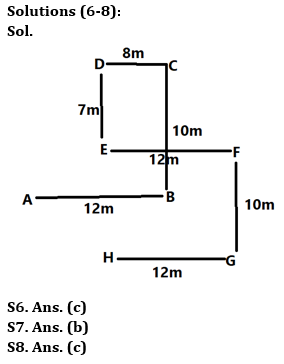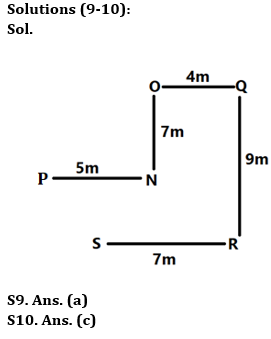Latest Banking jobs   »

# Reasoning Quizzes Quiz For IRDA AM 2023-27th May

Directions (1-5): In these questions, relationship between different elements is shown in the statements. The statements are followed by conclusions. Study the conclusions based on the given statements and select the appropriate answer. Give answer-
(a) If only conclusion II is true
(b) If only conclusion I is true
(c) If both conclusions I and II are true
(d) If either conclusion I or II is true
(e) If neither conclusion I nor II is true

Q1. Statements: A > G < J, I ≤ D, J < O = K > D
Conclusions: I. G > D II. O > I

Q2. Statements: P ≥ W ≤ Z, W > K, Q > O < Z
Conclusions: I. W > Q II. Z > K

Q3. Statements: B ≥ L < J > O, E > Y < L
Conclusions: I. B > Y II. J > Y

Q4. Statements: T > O < A < Q ≥ M > N < V
Conclusions: I. T > N II. A > M

Q5. Statements: B > Q ≥ R, Z > T ≥ R > J
Conclusions: I. B > J II. Z > Q

Directions (6-8): Answer the following question based on the information given below:
Eight people (A, B, C, D, E, F, G & H) sit in certain arrangement in the park. A sits 12m to the west of B. F sits 12m to the east of E. D sits 7m to the north of E. C sits 8m to the east of D. G sits 10m to the south of F. H sits 12m to the west of G. C sits 10m to the north of B.

Q6. In which direction is C with respect to G?
(a) North east
(b) South east
(c) North west
(d) South west
(e) None of the above

Q7. What is the shortest distance between F and B?
(a) 4m
(b) 5m
(c) 6m
(d) 7m
(e) None of the above

Q8. What is the shortest distance between D and H?
(a) 15m
(b) 16m
(c) 17m
(d) 18m
(e) None of the above

Directions (9-10): Answer the following question based on the information given below:
Rudraksh started walking from point P in east direction. After walking 5m he turns left from point N and walks for 7m till point O. He then turns right and walks 4m till point Q. He then turns right and walks for 9m till point R and then turns right and walks 7m till point S.

Q9. In which direction is point Q with respect to point S?
(a) North east
(b) South east
(c) North west
(d) South west
(e) None of the above

Q10. In which direction is point O with respect to point R?
(a) North east
(b) South east
(c) North west
(d) South west
(e) None of the above

Solutions

Solutions (1-5):
S1. Ans. (a)
Sol. I. G>D (False) II. O>I (True)
S2. Ans. (a)
Sol. I. W>Q(False) II. Z>K (True)
S3. Ans. (c)
Sol. I. B>Y(True) II. J>Y (True)
S4. Ans. (e)
Sol. I. T>N (False) II. A>M (False)
S5. Ans. (b)
Sol. I. B > J (True) II. Z > Q (False)## FAQs

### How many sections are there in the IRDAI exam?

There are 4 sections in the IRDAI assistant manager online preliminary exam. They are Reasoning, English Language, General awareness, Quantitative aptitude.

#### Congratulations!Union Budget 2023-24: Free PDF